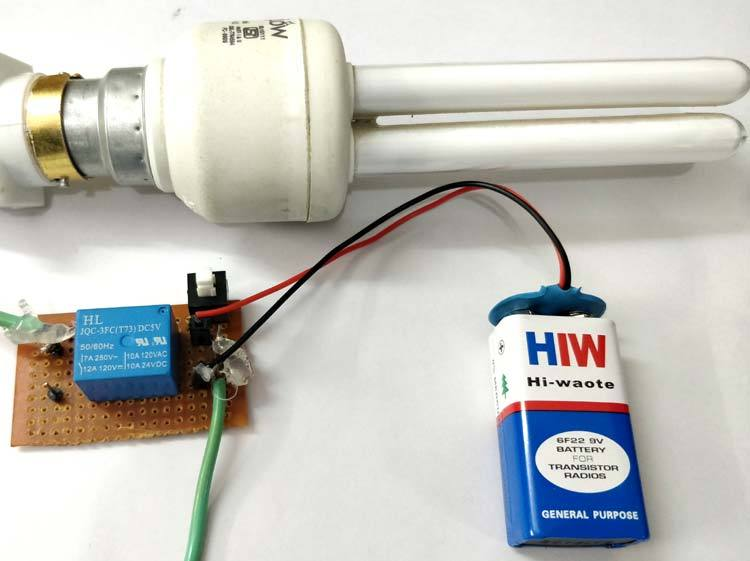# Simple Relay CircuitLet’s take a simple exampl…Simple Relay Circuit

Let’s take a simple example where we will be turning on an AC lamp (CFL) by using a relay switch. In this relay circuit we use a push button to trigger a 5V relay, which in turn, complete the second circuit and turn on the lamp.

Find the circuit details here: https://circuitdigest.com/electronic-circuits/simple-relay-switch-circuit-diagram